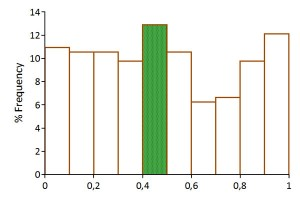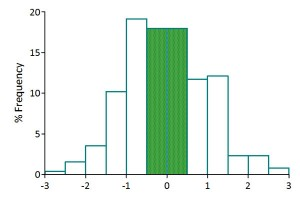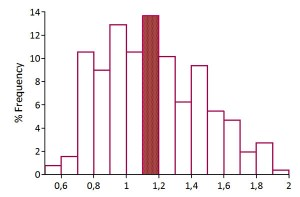# Plans based on average assumptions ……

This entry is part 3 of 4 in the series The fallacies of scenario analysis

The Flaw of Averages states that: Plans based on the assumption that average conditions will occur are usually wrong. (Savage, 2002)

Many economists use what they believe to be most likely1 or average values2  (Timmermann, 2006) (Gavin & Pande, 2008) as input for the exogenous variables in their spreadsheet calculations.

We know however that:

1. the probability for any variable to have outcomes equal to any of these values is close to zero,
1. and that the probability of having outcomes for all the (exogenous) variables in the spreadsheet model equal to their average is virtually zero.

So why do they do it? They obviously lack the necessary tools to calculate with uncertainty!

But if a small deviation from the most likely value is admissible, how often will the use of a single estimate like the most probable value be ‘correct’?

We can try to answer that by looking at some probability distributions that may represent the ‘mechanism’ generating some of these variables.

Let’s assume that we are entering into a market with a new product, we know of course the maximum upper and lower limit of our future possible market share, but not the actual number so we guess it to be the average value = 0,5. Since we have no prior knowledge we have to assume that the market share is uniformly distributed between zero and one:If we then plan sales and production for a market share between 0, 4 and 0, 5 – we would out of a hundred trials only have guessed the market share correctly 13 times. In fact we would have overestimated the market share 31 times and underestimated it 56 times.

Let’s assume a production process where the acceptable deviation from some fixed measurement is 0,5 mm and where the actual deviation have a normal distribution with expected deviation equal to zero, but with a standard deviation of one:Using the average deviation to calculate the expected error rate will falsely lead to us to believe it to be zero, while it in fact in the long run will be 64 %.

Let’s assume that we have a contract for drilling a tunnel, and that the cost will depend on the hardness of the rock to be drilled. The contract states that we will have to pay a minimum of \$ 0.5M and a maximum of \$ 2M, with the most likely cost being \$ 1M. The contract and our imperfect knowledge of the geology make us assume the cost distribution to be triangular:Using the average3 as an estimate for expected cost will give a correct answer in only 14 out of a 100 trials, with cost being lower in 45 and higher in 41.

Now, let’s assume that we are performing deep sea drilling for oil and that we have a single estimate for the cost to be \$ 500M. However we expect the cost deviation to be distributed as in the figure below, with a typical small negative cost deviation and on average a small positive deviation:So, for all practical purposes this is considered as a low economic risk operation. What they have failed to do is to look at the tails of the cost deviation distribution that turns out to be Cauchy distributed with long tails, including the possibility of catastrophic events:The event far out on the right tail might be considered a Black Swan (Taleb, 2007), but as we now know they happen from time to time.

So even more important than the fact that using a single estimate will prove you wrong most of the times it will also obscure what you do not know – the risk of being wrong.

Don’t worry about the average, worry about how large the variations are, how frequent they occur and why they exists. (Fung, 2010)

Rather than “Give me a number for my report,” what every executive should be saying is “Give me a distribution for my simulation.”(Savage, 2002)

### References

Gavin,W.,T. & Pande,G.(2008), FOMC Consensus Forecasts, Federal Reserve Bank of St. Louis Review, May/June 2008, 90(3, Part 1), pp. 149-63.

Fung, K., (2010). Numbers Rule Your World. New York: McGraw-Hill.

Savage, L., S.,(2002). The Flaw of Averages. Harvard Business Review, (November), 20-21.

Savage, L., S., & Danziger, J. (2009). The Flaw of Averages. New York: Wiley.

Taleb, N., (2007). The Black Swan. New York: Random House.

Timmermann, A.,(2006).  An Evaluation of the World Economic Outlook Forecasts, IMF Working Paper WP/06/59, www.imf.org/external/pubs/ft/wp/2006/wp0659.pdf

### Endnotes

Series Navigation<< Public Works ProjectsYou only live once >>
1. Most likely estimates are often made in-house based on experience and knowledge about their operations. []
2. Forecasts for many types of variable can be bought from suppliers of ‘consensus forecasts’. []
3. The bin containing the average in the histogram. []